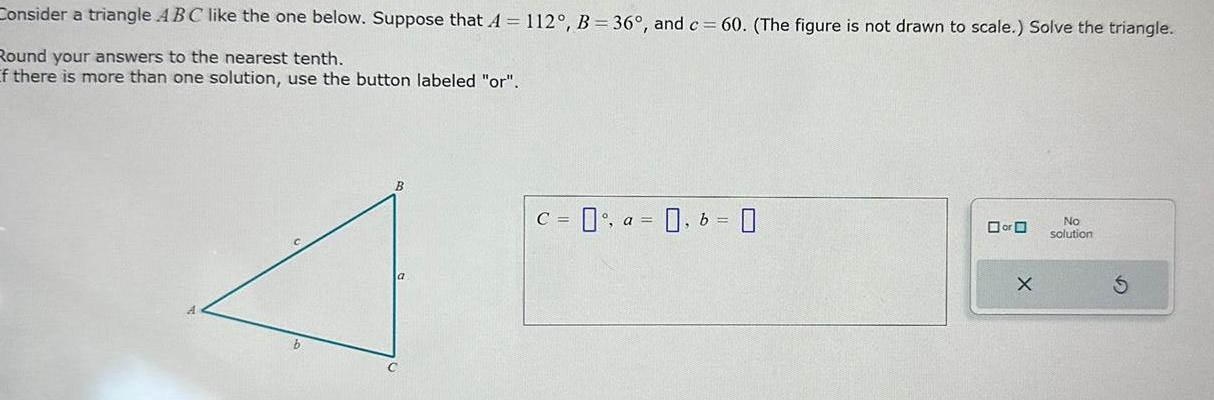Question:

# Consider a triangle ABC like the one below Suppose that A

Last updated: 9/19/2023Consider a triangle ABC like the one below Suppose that A 112 B 36 and c 60 The figure is not drawn to scale Solve the triangle Round your answers to the nearest tenth If there is more than one solution use the button labeled or C a b 0 or X No solution